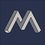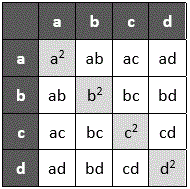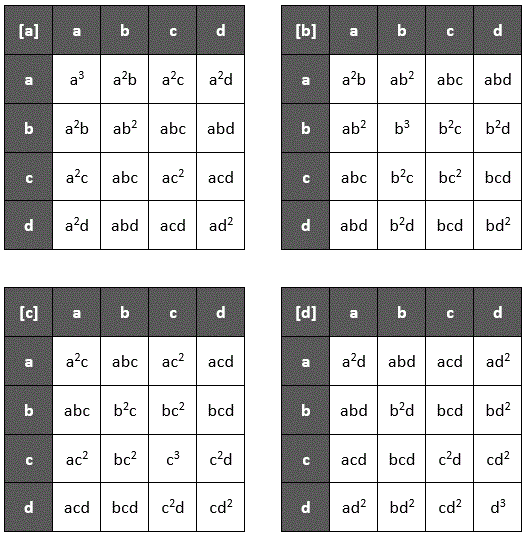# Sum of squares

Can anyone share a proof or a reference for the following property

$(a + b + c + d + \ldots)^2 = (a^2 + b^2 + c^2 + \ldots ) + 2(ab + bc + cd \ldots )$

$\bigg(\sum {a}\bigg)^2 = \sum {a^2} + 2\sum {ab}$

$\sum {a}$ means cyclicNote by Mahdi Raza
1 year, 1 month ago

This discussion board is a place to discuss our Daily Challenges and the math and science related to those challenges. Explanations are more than just a solution — they should explain the steps and thinking strategies that you used to obtain the solution. Comments should further the discussion of math and science.

When posting on Brilliant:

• Use the emojis to react to an explanation, whether you're congratulating a job well done , or just really confused .
• Ask specific questions about the challenge or the steps in somebody's explanation. Well-posed questions can add a lot to the discussion, but posting "I don't understand!" doesn't help anyone.
• Try to contribute something new to the discussion, whether it is an extension, generalization or other idea related to the challenge.

MarkdownAppears as
*italics* or _italics_ italics
**bold** or __bold__ bold
- bulleted- list
• bulleted
• list
1. numbered2. list
1. numbered
2. list
Note: you must add a full line of space before and after lists for them to show up correctly
paragraph 1paragraph 2

paragraph 1

paragraph 2

[example link](https://brilliant.org)example link
> This is a quote
This is a quote
    # I indented these lines
# 4 spaces, and now they show
# up as a code block.

print "hello world"
# I indented these lines
# 4 spaces, and now they show
# up as a code block.

print "hello world"
MathAppears as
Remember to wrap math in $$ ... $$ or $ ... $ to ensure proper formatting.
2 \times 3 $2 \times 3$
2^{34} $2^{34}$
a_{i-1} $a_{i-1}$
\frac{2}{3} $\frac{2}{3}$
\sqrt{2} $\sqrt{2}$
\sum_{i=1}^3 $\sum_{i=1}^3$
\sin \theta $\sin \theta$
\boxed{123} $\boxed{123}$

Sort by:

This isn't true. Set $a=b=1$. You get $( 1 + 1)^2 = (1^2 + 1^2) + (1 \times 1)$, which is obviously false.

- 1 year, 1 month ago

What about $n > 1$?

- 1 year, 1 month ago

What about it? I've shown a counterexample. What else is there?

- 1 year, 1 month ago

My question was $a = b > 1$.

- 1 year, 1 month ago

Just try $a=b=2$. What do you get?

- 1 year, 1 month ago

$16 \neq 12$

I have now understood. I'll tell @Mahdi Raza

- 1 year, 1 month ago

No no no.. I've added a clarification of what i meant @Pi Han Goh

- 1 year, 1 month ago

Yup, that's what I assumed. My answer still holds.

- 1 year, 1 month ago

Whoops.. Sorry @Pi Han Goh and @Yajat Shamji.. Forgot a 2 in there

- 1 year, 1 month ago

- 1 year, 1 month ago

@Zakir Husain, @Mahdi Raza needs your help...

- 1 year, 1 month ago

@Yajat Shamji, @Mahdi Raza - It is easy to prove, I'll write a note on it's proof. Just wait for sometime!

- 1 year, 1 month ago

I said I'm out!

- 1 year, 1 month ago

@Mahdi Raza- I have proved the equation (in rough not on brilliant) : $(\sum_{i=0}^{j}a_i)^p=\sum_{b_0=0}^{j}\sum_{b_1=0}^{j}\sum_{b_2=0}^{j}...\sum_{b_{p-1}=0}^{j}a_{b_0}a_{b_1}a_{b_2}...a_{b_{p-1}}$ where $p \in Z$

- 1 year, 1 month ago

If you put $p=2$ you get

$\sum_{b_0=0}^{j}\sum_{b_1}^{j}a_{b_0}a_{b_1}=a_0^2+a_0a_1+a_0a_2...+a_0a_j+a_1a_0+a_1^2+a_1a_2+a_1a_3...+a_1a_j+a_2a_0+a_2a_1+a_2^2+a_2a_3...=(a_0^2+a_1^2+a_2^2...a_j^2)+(a_0a_1+a_0a_2...+a_0a_j+a_1a_0+a_1a_2+a_1a_3...+a_1a_j...)=\sum_{i=0}^{j}a_i^2+\sum_{k=0}^{j}\sum_{l=0}^{j}a_ka_l$

- 1 year, 1 month ago

@Mahdi Raza see here

- 1 year, 1 month ago

Hmm.. it seems very elaborative but it's hard to see/understand.

- 1 year, 1 month ago

Here's a visual that might help you see how the property works:The grid shows the multiplication of $(a + b + c + d)$ with $(a + b + c + d)$, and each term is put as a row or column header. Each cell in the grid is then the product of its row and column header.

There is reflective symmetry along the diagonal of the grid, so each term along the diagonal ($a^2$, $b^2$, $c^2$, and $d^2$) appears once, and each of the other terms ($ab$, $ac$, $ad$, $bc$, $bd$, and $cd$) appears twice because they have a reflection.

- 1 year, 1 month ago

Lovely proof!! Thanks for sharing. This is by far the most easiest I’ve seen.

- 1 year, 1 month ago

@David Vreken, i was thinking whether something similar can be created for:

$(a + b + c + d + \ldots)^3$

$\bigg(\sum {a}\bigg)^3$

$\sum {a}$ means cyclic

- 1 year, 1 month ago

@Mahdi Raza see my comments, there i proved an equation for any power $p$

- 1 year, 1 month ago

You can show that using a cube, but it starts to get large and cumbersome. Here are the $4$ different layers of the $4 \times 4 \times 4$ cube showing $(a + b + c + d)^3$:which shows that:

$(a + b + c + d + ...)^3 = (a^3 + b^3 + c^3 + d^3 + ...) + 3(a^2b + a^2c + a^2d + ab^2 + b^2c + b^2d + ...) + 6(abc + abd + acd + bcd + ...)$

$(\sum a)^3 = \sum a^3 + 3 \sum a^2b + 6 \sum abc$

Unfortunately, trying to show a fourth power or higher using this method is extremely complicated because it needs more than three dimensions.

- 1 year, 1 month ago

You don't need to do it for more big numbers see my comments it is for general power

- 1 year, 1 month ago

Yes, I saw that, and it is very good, but I was answering @Mahdi Raza's question if a similar method (of using the grid) can be created for $(a + b + c + d + ...)^3$.

- 1 year, 1 month ago

@Zakir Husain, can you provide a proof for your general-power solution?

- 1 year, 1 month ago

Yes I'll but before that I'm more interested in areas of different regions remembered this question

- 1 year, 1 month ago

Actually, the preface of that daily challenge has the answer. Can you access the archive? If not I'll help you with a screenshot for that

- 1 year, 1 month ago

See my new note here also try the Bonus given in the last.

- 1 year, 1 month ago

@David Vreken, Superb!! Yeah you're right, we would need 3D visualization which is indeed cumbersome (and messy as well to show so many terms). Thanks for proving these!! Can I use this idea to make a video for $\bigg(\sum {a}\bigg)^2$ and $\bigg(\sum {a}\bigg)^3$, in case i make one.

- 1 year, 1 month ago

I assume you mean the YouTube Channel with some of my math lectures? I made that for my students when we had to switch to online learning for the quarantine. There's nothing dazzling (like animations) on it, but just some good basic high school math lessons. :-)

- 1 year, 1 month ago

Yeah this one. It's good, i watched one or two.

And, Can I use this idea to make a video for $\bigg(\sum {a}\bigg)^2$ and $\bigg(\sum {a}\bigg)^3$, in case i make one.

- 1 year, 1 month ago

I hope you are enjoying the puns, too!

Yes, you can use that idea to make a video. It's not original to me, anyway, and at least the two-dimensional grid is a method I found in some textbook (I can't remember which one, though).

- 1 year, 1 month ago

Yeah

"Do you think this is right?"

"I don't know, is it 90 degrees" lol

- 1 year, 1 month ago

ha ha classic

- 1 year, 1 month ago

By the way, I sometimes get a notification when you comment on an old daily challenge problem, but since I don't have a Premium account I can't read them if the daily challenge problem is expired (they usually expire after about a week). So I'm sorry that I'm not responding to those comments!

- 1 year, 1 month ago

Ohh, totally forgot about that. No problem at all, I was just commenting on how great (actually BRILLIANT) your solutions were. Especially those with the animations. I was not on brilliant for a year so i was exploring the archives section. Pretty great work done only on M.S. paint by you. Thanks for making those!

- 1 year, 1 month ago